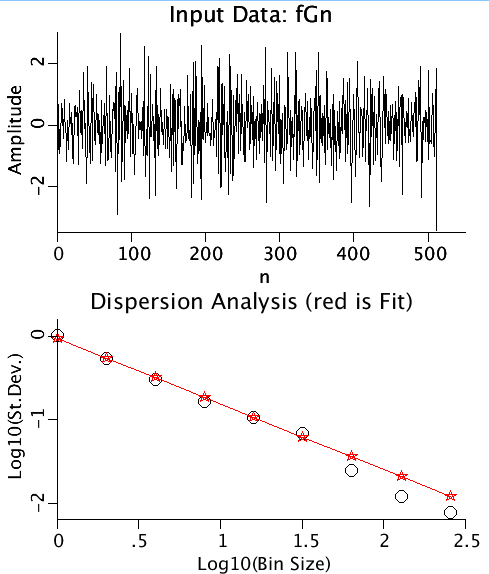# DISP

Model number
0353

Computes the Hurst coefficient for a fractional Gaussian noise series by binning the data (averaging adjacent points) and calculating the standard deviation as a function of bin size.

## Description

```   Dispersion Analysis:

Let x(i), i=1,2,...n be a series of n points. Calculate the standard
deviation. Next take the numbers in groups (bins) of two and average
them (i.e., x2(1)=.5*(x(1)+x(2)), x2(2)=.5*(x(3)+x(4)), ... x2(N/2)=
0.5*(x(N-1)+x(N)). Calculate the standard deviation of this new
series. Continue to double the bin size and repeat this process until
the bin size reaches n/2. If the OVERLAP option is used, the averages
become x2(1)=.5*(x(1)+x(2)), x2(2)=.5*(x(2)+x(3)), ... x2(N/2)=
0.5*(x(N-1)+x(N)).

The logarithms of the bin sizes (abscissa) versus the logarithms of
the standard deviations is approximately linear, and the slope equals
the estimated Hurst coefficient minus 1.0.

It is customary to omit the three largest bins from the analysis
because they are based on the standard deviations of fewer points:
If a series contained 512 points, and the OVERLAP option was turned off
the standard deviation for bin size 256 would be based on two points,
for bin size 128, 4 points, and for bin size 64, 8 points.

The model contains three curves for dispersional analysis,
generated by FGP, the fractional Gaussian Process model. The
curves were generated with Hurst coefficients of 0.2, 0.5, and 0.7,
coresponding to anti-correlated noise, white noise, and correlated
noise.
```Upper Panel: A time series of Gaussianly distributed random numbers with mean=0, variance=4.
Lower Panel: The autocovariance function (black) and the autocorrelation function (red) are plotted as functions of the lag.

## Equations

See Description above.

The equations for this model may be viewed by running the JSim model applet and clicking on the Source tab at the bottom left of JSim's Run Time graphical user interface. The equations are written in JSim's Mathematical Modeling Language (MML). See the Introduction to MML and the MML Reference Manual. Additional documentation for MML can be found by using the search option at the Physiome home page.

Key terms
dispersion
fractal
time series
fGn
fractional Gaussian noise
Hurst
Acknowledgements

Please cite https://www.imagwiki.nibib.nih.gov/physiome in any publication for which this software is used and send one reprint to the address given below:
The National Simulation Resource, Director J. B. Bassingthwaighte, Department of Bioengineering, University of Washington, Seattle WA 98195-5061.

Model development and archiving support at https://www.imagwiki.nibib.nih.gov/physiome provided by the following grants: NIH U01HL122199 Analyzing the Cardiac Power Grid, 09/15/2015 - 05/31/2020, NIH/NIBIB BE08407 Software Integration, JSim and SBW 6/1/09-5/31/13; NIH/NHLBI T15 HL88516-01 Modeling for Heart, Lung and Blood: From Cell to Organ, 4/1/07-3/31/11; NSF BES-0506477 Adaptive Multi-Scale Model Simulation, 8/15/05-7/31/08; NIH/NHLBI R01 HL073598 Core 3: 3D Imaging and Computer Modeling of the Respiratory Tract, 9/1/04-8/31/09; as well as prior support from NIH/NCRR P41 RR01243 Simulation Resource in Circulatory Mass Transport and Exchange, 12/1/1980-11/30/01 and NIH/NIBIB R01 EB001973 JSim: A Simulation Analysis Platform, 3/1/02-2/28/07.